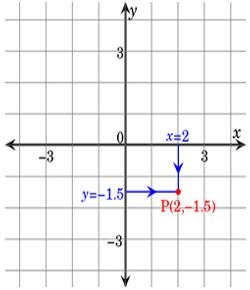# Math Quiz: Take This Coordinate Geometry Questions!

23 Questions | Attempts: 2735Settings• 1.
The mid point of the line joining (a,-b) and (3a,5b) is
• A.

(2a,2b)

• B.

(-a,2b)

• C.

(2a,4b)

• D.

(-a,-3b)

• 2.
The point P  which divides the line segment joining the points  A(1,-3) and B(-3,9)   internally  in the ratio 1:3 is
• A.

(0,0)

• B.

(2,1)

• C.

(5/3,2)

• D.

(1,-2)

• 3.
If the line segment joining the point A(3,4) and B(14,-3)meets the x-axis at P,then the ratio in which P divides the segment AB is
• A.

4:3

• B.

3:4

• C.

2:3

• D.

4:1

• 4.
The centroid of the triangle with vertices at (-2,-5),(-2,12)and (10,-1)is
• A.

(2,2)

• B.

(6,6)

• C.

(4,4)

• D.

(3,3)

• 5.
If (1,2), (4,6), (x,6) and (3,2) are the vertices of a parallelogram taken in order, Then the value of x is
• A.

6

• B.

2

• C.

1

• D.

3

• 6.
Area of the triangle formed by the points (1,1)        , (0,1) , (0,0)       and (1,0) is
• A.

2 sq.units

• B.

4 sq.units

• C.

1 sq.units

• D.

3 sq.units

• 7.
Area of a quadrilateral formed by the points (1,1), (0,1), (0,0) and (1,0) is
• A.

1 sq.units

• B.

4 sq.units

• C.

2 sq.units

• D.

3 sq.units

• 8.
The angle of inclination of a straight  line parallel to X-axis is
• A.

0

• B.

60

• C.

45

• D.

90

• 9.
Slope of the line joining the points (3,-2) and (-1,a)   is - 3/2  , then the value of a
• A.

4

• B.

3

• C.

2

• D.

1

• 10.
Slope of the straight line which is perpendicular to  the straight line joining the points (-2,6) and (4,8) is
• A.

-3

• B.

1/3

• C.

3

• D.

- 1/3

• 11.
The points of intersection of the straight line 9x-y-2=0 and 2x+y-9=0 is
• A.

(1,7)

• B.

(-1,-7)

• C.

(7,1)

• D.

(-1,7)

• 12.
The straight line 4x+3y-12=0 intersects they-axis at
• A.

(0,4)

• B.

(3,0)

• C.

(3,4)

• D.

(0,-4)

• 13.
The slope of the straight line 7y-2x=11 is equal to
• A.

2/7

• B.

-7/2

• C.

7/2

• D.

2/7

• 14.
The equation of a straight line passing through the point (2,-7) and  Parallel to x-axis is
• A.

Y=-7

• B.

X=2

• C.

X=-7

• D.

Y=2

• 15.
The x and y-intercepts of the line 2x-3y+6=0,respectively are
• A.

-3,2

• B.

2,3

• C.

3,2

• D.

3,-2

• 16.
The Centre  of a circle is (-6,4).If one end of the diameter of the    Circle is at (-12,8),then the other end is at
• A.

(0,0)

• B.

(-18,12)

• C.

(-9,6)

• D.

(-3,2)

• 17.
The equation of the straight line passing  through the origin and   Perpendicular to   the straight line 2x+3y-7=0
• A.

3x-2y=0

• B.

2x+3y=0

• C.

Y+5=0

• D.

Y-5=0

• 18.
The equation of a straight line parallel to y-axis and passing through the  point (-2,5) is
• A.

X+2=0

• B.

X-2=0

• C.

Y+5=0

• D.

Y-5=0

• 19.
If the  point (2,5) ,(4,6) and (a, a) are collinear, then the  value of a is equal to
• A.

8

• B.

-8

• C.

4

• D.

-4

• 20.
If a straight line y=2x +k passes through the point (1,2), then the value of   k is equal to
• A.

0

• B.

4

• C.

5

• D.

-3

• 21.
The equation of a straight line having slope 3 and y-intercept-4 is
• A.

3x-y-4=0

• B.

3x+y-4=0

• C.

3x-y+4=0

• D.

3x+y+4=0

• 22.
The point of intersection of the straight lines y=0 and x=-4 is
• A.

(-4,0)

• B.

(0,-4)

• C.

(0,4)

• D.

(4,0)

• 23.
The value of k if the straight lines 3x+6y+7=0 and 2x+k y=5 are perpendicular is
• A.

-1

• B.

1

• C.

2

• D.

1/2

## Related TopicsBack to top
×

Wait!
Here's an interesting quiz for you.